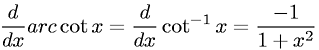Equations > Calculus > Differentiation/Differentals > Derivative of Inverse Cotangent (Arccotangent)

### Derivative of Inverse Cotangent (Arccotangent)Latex Code:

MathML Code:

 $\fracd\mathrm{dx}\mathrm{arc}\mathrm{cot}x=\fracd\mathrm{dx}{\mathrm{cot}}^{-1}x=\frac-11+{x}^{2}$

MathType 5.0: